# tkinter.ttk的Treeview组件超详细讲解

### 以一个源码案例讲解Treeview组件的结构

```from tkinter import *
from tkinter.ttk import *

root = Tk()

tree1 = Treeview(root, columns=('qy', 'dz'))
# 创建树表格组件，栏目有3个：#0, qy, dz

tree1.column('#0', width=90, anchor=CENTER,stretch=False)
tree1.column('qy', width=90, anchor=CENTER)
tree1.column('dz', width=160, anchor=CENTER)
# 定义3个栏目的宽度，对齐方法，宽度是否窗体变化

# 定义3个栏目的表头文字

sf1 = tree1.insert('', END, text='广东',open=True)
sf2 = tree1.insert('', END, text='湖南',open=True)
# 在根节点‘’下添加2个子节点：广东，湖南

tree1.insert(sf1, END, text='广州市',values=('海珠区','阅江中路380号'))
tree1.insert(sf1, END, text='深圳市',values=('南山区','华侨城侨香路11号'))
tree1.insert(sf1, END, text='东莞市',values=('南城区','元美东路3号济亨网'))
# 在广州（sf1）节点下，插入3条记录：#0栏 = text,其它栏 = values()

tree1.insert(sf2, END, text='长沙市',values=('雨花区','韶山中路108号'))
tree1.insert(sf2, END, text='湘潭市',values=('岳塘区','书院路42号云峰工作室'))
tree1.insert(sf2, END, text='衡阳市',values=('蒸湘区','祝融路名都花园B9栋107室'))
# 在湖南（sf2）节点下，插入3条记录：#0栏 = text,其它栏 = values()

tree1.insert(sf2, END, text='长沙市',values=('岳麓区','梅溪湖路复兴小区709号'))
tree1.insert(sf1, END, text='广州市',values=('白云区','下塘西路545号'))
# 以同样方法插入2条记录，它们会根据父节点找到自己的位置

tree1.pack(fill=BOTH,expand=True)

root.mainloop()```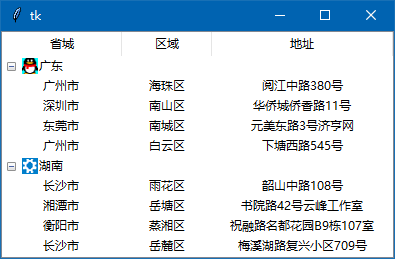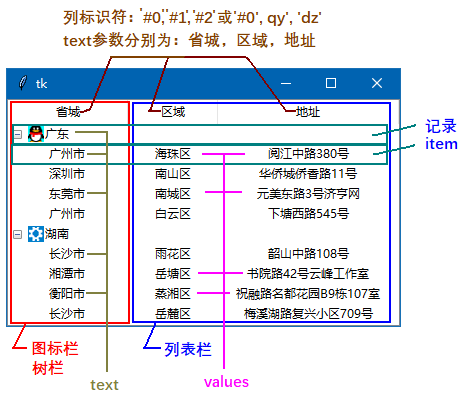Treeview组件做为一个整体，可以看成是树形组件和列表组件相合的一个组件。左边第1列就是树栏，第1列外，右边的列都是列表栏。

Treeview组件也可以只显示树栏，参数：show=’tree’

Treeview组件做为一个整体，每一行是一条记录，Treeview组件称一行为item，但每一行的“文本”在树栏和列表栏是不同的参数：在树栏，item的文本参数是：text，在列表栏的文本参数是：values，values这是一个列表或元组，以上图为例：

Treeview组件也有自己的标题栏，组件最上面的“省城，区域，地址”就是标题栏上每一列的名称，这些名称不再区分，都是用参数text来表示（只有在每一行的记录中[item]，才区别text，values）

“省城，区域，地址”是显示给用户看的，编程时，如何标识每一列呢？这有2种方法：

### 做一个纯树栏（只有图标栏）的Treeview组件

 参数 说明 columns 值为一个列表，列表中元素为列表标识符 displaycolumns 值为列表，元素是列表标识符，表示显示的列表顺序 height 表示要显示的行数 padding 组件四周的填充要素（我前面的文章有讲解） selectmode 选项: extended 选择多行（Ctrl+鼠标）；browse 只能选择一行none 不能改变选择；默认是选择多行 show 选项: tree 只显示树栏，headings 只显示除第一列的其它列tree headings 表示显示所有列

`tree1 = Treeview(root, columns=('qy', 'dz'))`

columns参数里只有2个列表标识符元素，但列表是有3个，因为第1个列表不用写入，它是固定存在的，不能取消，不能删除。（“#0”是第1列的标识符）

`tree1 = Treeview(root,show='tree')`

Treeview组件创建好了，现在就要开始创建节点，根节点已经存在了，它用一个空字符''来表示。

```img1=PhotoImage(file='16-1.png')
img2=PhotoImage(file='16-2.png')```

insert (父组件，插入位置，text=’节点文本image=图像对象，open=/)

注：open参数为True表示分层的各节点是展开的，为False则折叠各节点。

插入位置跟以前学的Text组件一样的。

```sf1 = tree1.insert('', END, text='广东',image=img1,open=True)
sf2 = tree1.insert('', END, text='湖南',image=img2,open=True)```

上面代码创建了sf1 和 sf2 这个节点，我们再在这2个节点下创建记(item)，主要是改父组件，text参数：

```tree1.insert(sf1, END, text='广州市')
tree1.insert(sf1, END, text='深圳市')
tree1.insert(sf1, END, text='东莞市')
# 在广州（sf1）节点下，插入3条子节点

tree1.insert(sf2, END, text='长沙市')
tree1.insert(sf2, END, text='湘潭市')
tree1.insert(sf2, END, text='衡阳市')
# 在湖南（sf2）节点下，插入3条子节点

tree1.insert(sf2, END, text='长沙市')
tree1.insert(sf1, END, text='广州市')
# 以同样方法插入2条记录，它们会根据父节点找到自己的位置```

```sf1-1=tree1.insert(sf1, END, text='广州市')
sf2-1=tree1.insert(sf2, END, text='长沙市')```

```from tkinter import *
from tkinter.ttk import *

root = Tk()

img1=PhotoImage(file='16-1.png')
img2=PhotoImage(file='16-2.png')

tree1 = Treeview(root,show='tree')

sf1 = tree1.insert('', END, text='广东',image=img1,open=True)
sf2 = tree1.insert('', END, text='湖南',image=img2,open=True)
# 在根节点‘’下添加2个子节点：广东，湖南

tree1.insert(sf1, END, text='广州市')
tree1.insert(sf1, END, text='深圳市')
tree1.insert(sf1, END, text='东莞市')
# 在广州（sf1）节点下，插入3条子节点

tree1.insert(sf2, END, text='长沙市')
tree1.insert(sf2, END, text='湘潭市')
tree1.insert(sf2, END, text='衡阳市')
# 在湖南（sf2）节点下，插入3条子节点

tree1.insert(sf2, END, text='长沙市')
tree1.insert(sf1, END, text='广州市')
# 以同样方法插入2条记录，它们会根据父节点找到自己的位置

tree1.pack(fill=BOTH,expand=True)

root.mainloop()```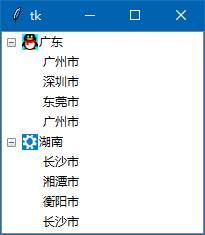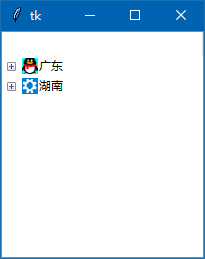### 做一个单列表栏的Treeview组件。

`tree1 = Treeview(root, columns=('qy', 'dz'),show='headings')`

```tree1.heading('qy', text='区域')

```tree1.heading('#1', text='区域')

‘#0’是固定给第1列，即树列的，现在它只是隐藏了而已。  丶丌皛

insert (父组件，插入位置，values=列表元组，image=图像对象)

`tree1.insert('', END, values=('海珠区','阅江中路380号'))`

```from tkinter import *
from tkinter.ttk import *

root = Tk() # 源码来自wb98.com

# 定义2个栏目的表头文字

tree1.insert('', END, values=('海珠区','阅江中路380号'))
tree1.insert('', END, values=('南山区','华侨城侨香路11号'))
tree1.insert('', END, values=('南城区','元美东路3号济亨网'))

tree1.insert('', END, values=('雨花区','韶山中路108号'))
tree1.insert('', END, values=('岳塘区','书院路42号云峰工作室'))
tree1.insert('', END, values=('蒸湘区','祝融路名都花园B9栋107室'))

tree1.insert('', END, values=('岳麓区','梅溪湖路复兴小区709号'))
tree1.insert('', END, values=('白云区','下塘西路545号'))
# 根节点（''）下，插入8条记录：#0栏 = text,其它栏 = values()

tree1.pack(fill=BOTH,expand=True)

root.mainloop()```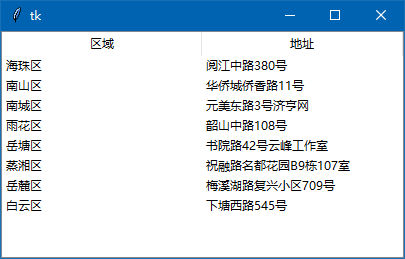`tree1.column('#0',width=0,stretch=False)`

cloumn()方法语法：

cloumn(列标识符，参数…….)

width : 列的宽度

anchor : 列内容的对齐方式

stretch : 布尔值，为真，列的宽度随窗体的宽度变化而变化，为假，则不会。

```from tkinter import *
from tkinter.ttk import *

root = Tk()  # 源码来自wb98.com

tree1 = Treeview(root, columns=('qy', 'dz'),show='tree')
tree1.column('#0',width=0,stretch=False)

tree1.insert('', END, values=('海珠区','阅江中路380号'))
tree1.insert('', END, values=('南山区','华侨城侨香路11号'))
tree1.insert('', END, values=('南城区','元美东路3号济亨网'))

tree1.insert('', END, values=('雨花区','韶山中路108号'))
tree1.insert('', END, values=('岳塘区','书院路42号云峰工作室'))
tree1.insert('', END, values=('蒸湘区','祝融路名都花园B9栋107室'))

tree1.insert('', END, values=('岳麓区','梅溪湖路复兴小区709号'))
tree1.insert('', END, values=('白云区','下塘西路545号'))
# 根节点（''）下，插入8条记录：#0栏 = text,其它栏 = values()

tree1.pack(fill=BOTH,expand=True)

root.mainloop()```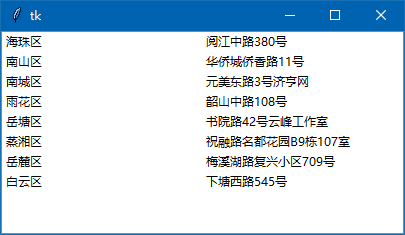### 做一个树栏和列表栏都显示的Treeview组件

下面就结合上面的知识，把一个Treeview组件所有列都显示出来。

```tree1.column('#0', width=120, anchor=CENTER)
tree1.column('qy', width=90, anchor=CENTER)
tree1.column('dz', width=180, anchor=CENTER)
# 定义3个栏目的宽度，对齐方法，宽度是否窗体变化```

```tree1.heading('#0', text='')

```sf1 = tree1.insert('', END, text='广东',image=img1,open=True)
sf2 = tree1.insert('', END, text='湖南',image=img2,open=True)```

接着，在sf1, sf2 2个子节点下，插入记录[item] ，插入一行记录内容时，要注意“父节点”要写对，插入的内容，textvalues2个参数都要写，（当然，textvalues也都可为空） values的元素如果少于列表数，则未赋值的列的数据为空，如果插入的数据多，多的数据则丢弃。

插入的记录[item]代码，以及全部代码在文章最前面就已经提供了。在这里就不再提供一次了。

关于Treeview组件结构以及分别创建纯树栏，纯列表栏，树栏和列表栏相合栏就讲到这里，下一篇文章我来讲讲Treeview组件的相关知识，如高，宽设置，图标的设置，记录的增加和修改和删除等。

此文章来自：wb98.com  网站还有相关的系列课程文章，感兴趣的可以前往。

<< 上一篇 下一篇 >>